• ## 华为机试刷题

千次阅读 2020-03-19 21:40:00
服务器广播 ...给出一个 N * N 数组，代表 N 个服务器， matrix[i][j] == 1 ，则代表 i 和 j 直接连接；不等于 1 时，代表 i 和 j 不直接连接。 matrix[i][i]== 1 ，即自己和自己直接连接。 matr...


服务器广播

题目：服务器连接方式包括直接相连，间接连接。 A 和 B 直接连接， B 和 c 直接连接，则 A 和 c 间接连接。直接连接和间接连接都可以发送广播。
给出一个 N * N 数组，代表 N 个服务器， matrix[i][j] == 1 ，则代表 i 和 j 直接连接；不等于 1 时，代表 i 和 j 不直接连接。 matrix[i][i]== 1 ，即自己和自己直接连接。 matrix[i][j]==matrix[j][i] 。计算初始需要给几台服务器广播，才可以使侮个服务器都收到广播。
输入描述： n * n 矩阵，
[[1,1,0],[1,1,0],[0,0,1]]
1
输出描述：整数
2
 int main () {

//vector<vector<int>> v{{1,0,1,0,1,1},{0,1,0,1,0,0},{1,0,1,0,0,0},{0,1,0,1,0,0},{1,0,0,0,1,0},{1,0,0,0,0,1}};
vector<vector<int>> v{{1,0,1,0,1,1},{0,1,0,0,0,0},{1,0,1,0,0,0},{0,0,0,1,0,0},{1,0,0,0,1,0},{1,0,0,0,0,1}};
unordered_map<int,set<int>> a;
auto len = v.size();
for (int i = 0;i<len;i++) {
for (int j=0;j<len;j++) {
if (v[i][j] == 1) {
a[i].insert(j);
}
}
}
for (auto iter = a.begin(); iter != a.end();iter++) {
int num = iter->first;
set<int> tmp =iter->second;
for (int j : tmp) {
if (num != j) {
a[j].insert(tmp.begin(),tmp.end());
}
}
}
set<set<int>> ret;
for (auto iter = a.begin(); iter != a.end();iter++) {
set<int> tmp =iter->second;
ret.insert(tmp);
}
cout << ret.size() << endl;

return 0;
}

心路历程：用什么数据结构有时比算法重要，数据结构也能体现解决方法。
开始拿到题目，纸上画了画人工是怎么思考的，第一感觉是想到做过的一道题，一个矩阵，某元素为1的话会像炸弹那样令跟它同一行和同一列的元素都变成1，新生成的1不会有这个效果。画格子画了会没啥感觉，又想到这题从描述就天然是个图问题，用dfs递归下？但是天然的对dfs比较排斥，估计写不来，并没有做过多思考。再画两下，突然就画出了题解中的数据结构，map, 接下来就比较容易了。中间也考虑过用两层vector，一想内层的大小不一致不能用, 题解中答案写完后试了下内层的vector也是可变的，大小能任意变，这题数据结构改成vector<vector> 应该也可以，第一层的下标就相当于map的key, 但是直观想内层的vector还得去重吧，改成vector<set> 估计会更好；还碰到对set中insert set 的迟疑，需要手动考虑去重吗？答案是不需要，暴力insert就行了。两个set怎么合并 ？ a.insert(b.begin(), b.end()) 即可。支持两层set吗？支持。怎么判定最后要多少个广播？不同的set个数。即相互连接的服务器的set最后里面的元素是一样的，为了保证能一样，所以用set没用unordered_set，而为了性能用了unordered_map。边界条件处理，这块得加强，没多少想法。如果有多个服务器每个都是孤岛，没跟外界连，是否最终会合并成一个？所以针对这种，要将v[i][i] 自己也放进set容器里
经验：
1.遇到报错一定要多检查括号写错没
ret.insert(tmp); 这句手误打成了 ret.insert< tmp >; 没检查出来，报错为：
reference to non-static member function must be called
2.对于迭代器使用不熟练，int num = iter->first; 写这块时不确定是
int num = *iter->first;
或者 int num = *（iter)->first）
或者 int num = *（iter->first）; 浪费了几分钟时间

首先是优先级要记牢：（优先级越高越先）
第一优先级：    ::   仅此一个作用域解析符
第二优先级：（）[] -> .
第三优先级：* &

迭代器：vector中 *iter就能访问到数据元素了，而map中不需要 * ，auto p = iter->first; 就能访问到第一个元素了

vector<int> bb{1,2,3,4};
for (auto i = bb.begin();i!=bb.end();i++) {
cout << *i << " ";
}

输出 ： 1 2 3 4

unordered_map<int,set<int>> a;
for (auto iter = a.begin(); iter != a.end();iter++) {
int num = iter->first;
set<int> tmp =iter->second;
}


判断一个string能否转成int数

string str = "12388sf";
int ret = 0;
to_int(str, ret);

bool to_int(const string& str, int& ret) {
int len = str.length();
std::string::size_type sz;
bool flag = false;
try {
ret = stoi(str,&sz);
if (sz == len) {
flag = true;
}
} catch (std::invalid_argument&) {
} catch (std::out_of_range&) { // {}内可以是空语句的
} catch (std::exception& e) {
cout << e.what() << endl;
}
//} catch (...) {
//}
return flag;
}


注意点：
stoi的原型为：
int stoi (const string&  str, size_t* idx = 0, int base = 10);
第二个参数为能转成数字的下一位的下标，是指针。第三个参数为要将第一个参数中数字用什么进制解释。如果str的第一个字符不是字符0-9，则会出异常，报 invalid_argument ， 如果范围超过了int，则报 out_of_range 异常，如果这两点都没问题，而 sz 的大小和 length 不相等，则说明数字后面还有非数字字符。所以这个函数用起来还是比较麻烦的，得自己check。
另一个用法举例：
std::string str_hex = "40c3";
int i_hex = std::stoi (str_hex,nullptr,16);
std::cout << str_hex << ": " << i_hex << '\n';

输出：
40c3:  16579


字符串分割

题目：实现字符串分割算法split(str,delim),传入待分割字符串和分割字符，返回数组，如果delim参数不传，则对字符串逐个分割。
比如调用split(“abcbebb”,‘b’)，返回为[“a”,“c”,“e”]，调用split(‘abcd’)，返回[‘a’,‘b’,‘c’,d’]
std::vector<std::string> split_string(const std::string& s, const char flag = ' ') {
if (s.empty()) {
return {};
}
std::vector<std::string> str_vec;
if (flag != '\0') { // 注意点一：用'\0'表示空字符，表示参数二没传入。这个一度阻塞了思路。同时记住'\0'很特殊，是ascii表的第一个，即是基准
istringstream iss(s);
std::string temp;
while (getline(iss, temp, flag)) {
if (!temp.empty()) {
str_vec.push_back(temp);
}
}
} else {
for(char i : s) {
string t;
t.push_back(i); // 注意点二：将char转成string的方法:往新申请的string里push_back(char)即可。另外注意不要用to_string，这个传入的参数是int float double等数字型的变量
str_vec.push_back(t);
}
}
return char_vec;
}


单词压缩编码

题目：给定一个单词列表，将这个列表编码成一个索引字符串 S 与一个索引列表 A。
例如，如果这个列表是 [“time”, “me”, “bell”]，将其表示为 S = “time#bell#” 和 indexes = [0, 2, 5]。
对于每一个索引，可以通过从字符串 S 中索引的位置开始读取字符串，直到 “#” 结束，来恢复之前的单词列表。
输入描述：数组。
输出描述：成功对给定单词列表进行编码的最小字符串长度
class Solution {
public:
int minimumLengthEncoding(vector<string>& words) {
sort(words.begin(), words.end(), [](const string& a, const string& b) -> bool {return a.length() > b.length();});
string ret = words + "#";
for (int i = 1; i < words.size(); ++i) {
auto found = ret.find(words[i] + "#");
if (found == string::npos) {
ret += words[i] + "#";
}
}
return ret.length();
}
};

思路很简单，按长度依次处理是关键，另外需要注意题目要求必须以“#”结束，所以比如 time# 可以匹配 me，不能匹配 ti, 为此将每个word加上“#”后才进行find。解法的时空效率不怎么高

打印任务排序

题目：某个打印机根据打印队列执行打印任务。打印任务分为九个优先级，分别采用数字1~9表示，数字越大优先级越高。打印机每次从队列头部取出第一个任务A，然后检查队列余下任务中有没有比A优先级更高的任务，如果有比A优先级高的任务，则将任务A放到队列尾部，否则执行任务A的打印。请编写一个程序，根据输入的打印队列，输出实际打印顺序
struct mydata{
int n;
int pos;
mydata(int i, int j):n(i),pos(j) {}
};

bool comp(const mydata& a, const mydata& b) {
return a.n < b.n;
}
int main () {
int n = 0;
while(cin>>n) {
vector<int> p;
deque<mydata> v;
for(int i=0;i<n;i++) {
int p = 0;
cin >> p;
mydata m(p,i);
v.push_back(m);
}
while(!v.empty()) {
auto m = *max_element(v.begin(), v.end(), comp);
int nn = m.n;
if (nn == v.front().n) {
p.push_back(m.pos);
v.pop_front();
} else {
auto t = v.front();
v.push_back(t);
v.pop_front();
}
}
for(auto i : p) {
cout << i << " ";
}
cout << endl;
}
return 0;
}

输入
6
1 1 9 1 1 1

输出
2 3 4 5 0 1


变量命名比较随意，机试先追求把题做出来，再追求命名、格式这些东西
deque 可以pop_front，vector没有
max_element 可以用于自定义对象，要自定义比较函数。同时有min_element，这两个的自定义比较函数中，对于数值型的统一用 return x < y; 如果需要取最小值，则用 min_element，如果要用最大值则用max_element。
同样的需要保留住原始输入的位置信息，所以创了个新数据类型


展开全文• 在线学习 import numpy as np """ 10X + 8 Y + 12 Z = 20 4X + 4Y + 2 Z = 8 2X -4 Y -2Z = -5 """ k_matrix = np.array([[10,8,12], [4,4,2], [2,-4,-2]]) ...result_matrix = np.array([,...

在线学习
import numpy as np

"""
10X + 8 Y + 12 Z = 20
4X + 4Y + 2 Z = 8
2X -4 Y -2Z = -5
"""
k_matrix = np.array([[10,8,12],
[4,4,2],
[2,-4,-2]])

result_matrix = np.array([,,[-5]])
x_matrix = np.linalg.solve(k_matrix,result_matrix)

print(x_matrix)


转载于:https://www.cnblogs.com/Frank99/p/11002401.html
展开全文• Mind Studio的是一套基于华为自研昇腾AI处理器开发的AI全栈开发工具平台，包括网络模型移植、应用开发、推理运行及自定义算子开发，通过Mind Studio能够进行工程管理、编译、性能分析等全流程开发，提高开发效率。...
一、Mind studio
Mind Studio的是一套基于华为自研昇腾AI处理器开发的AI全栈开发工具平台，包括网络模型移植、应用开发、推理运行及自定义算子开发，通过Mind Studio能够进行工程管理、编译、性能分析等全流程开发，提高开发效率。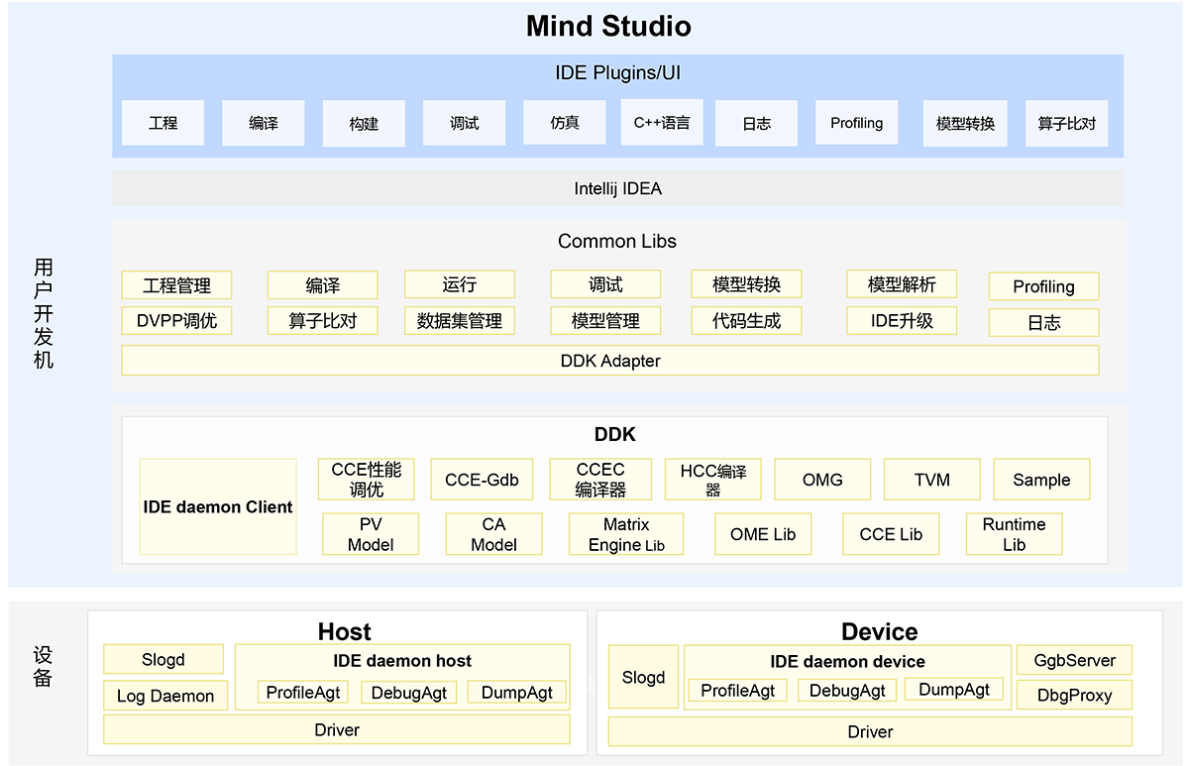模型管理工具-OMG/OME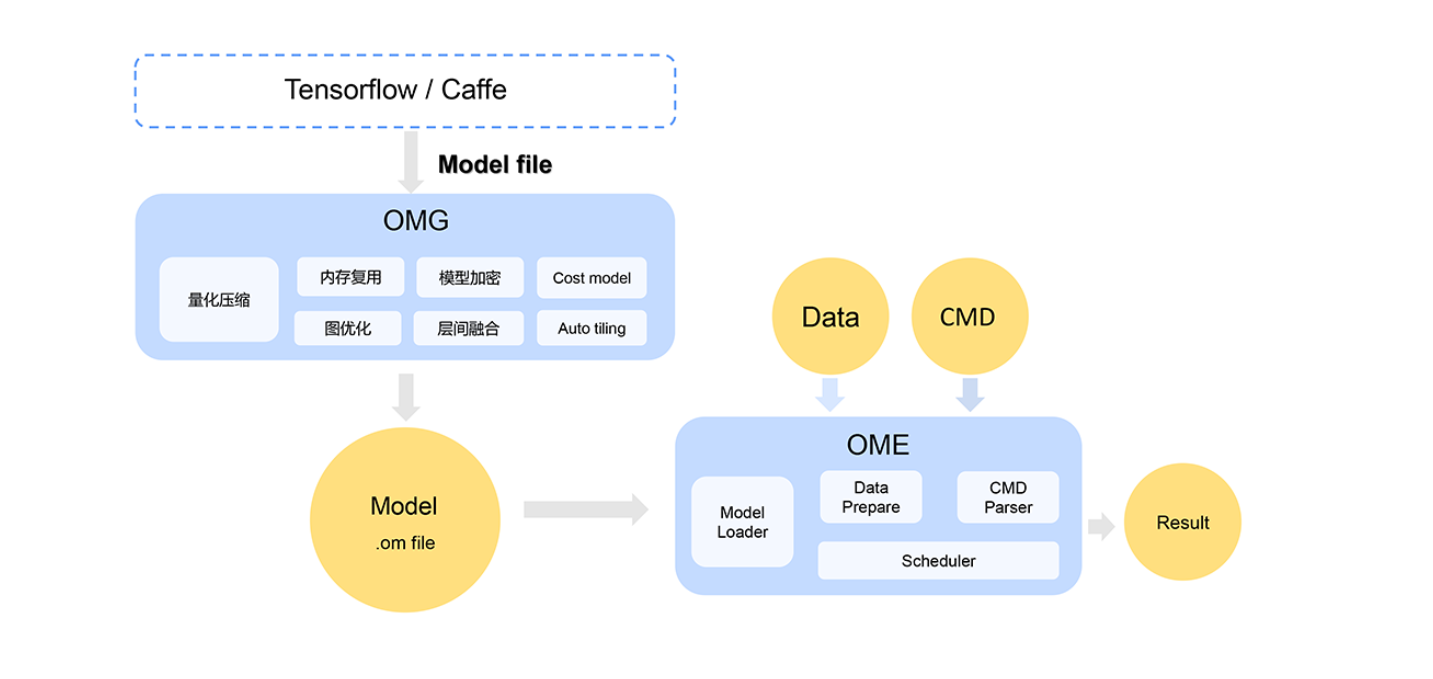业务编排工具-Matrix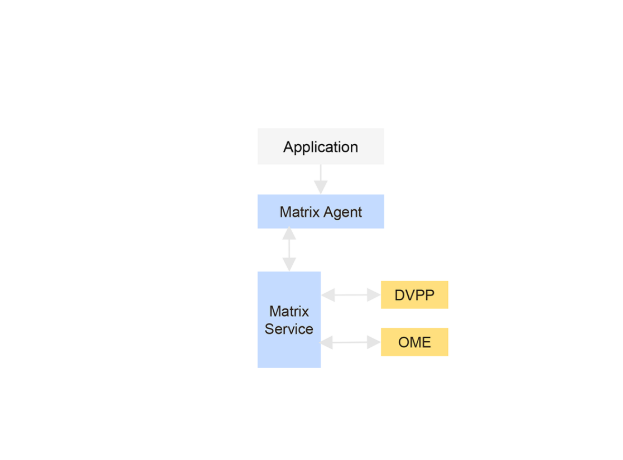日志工具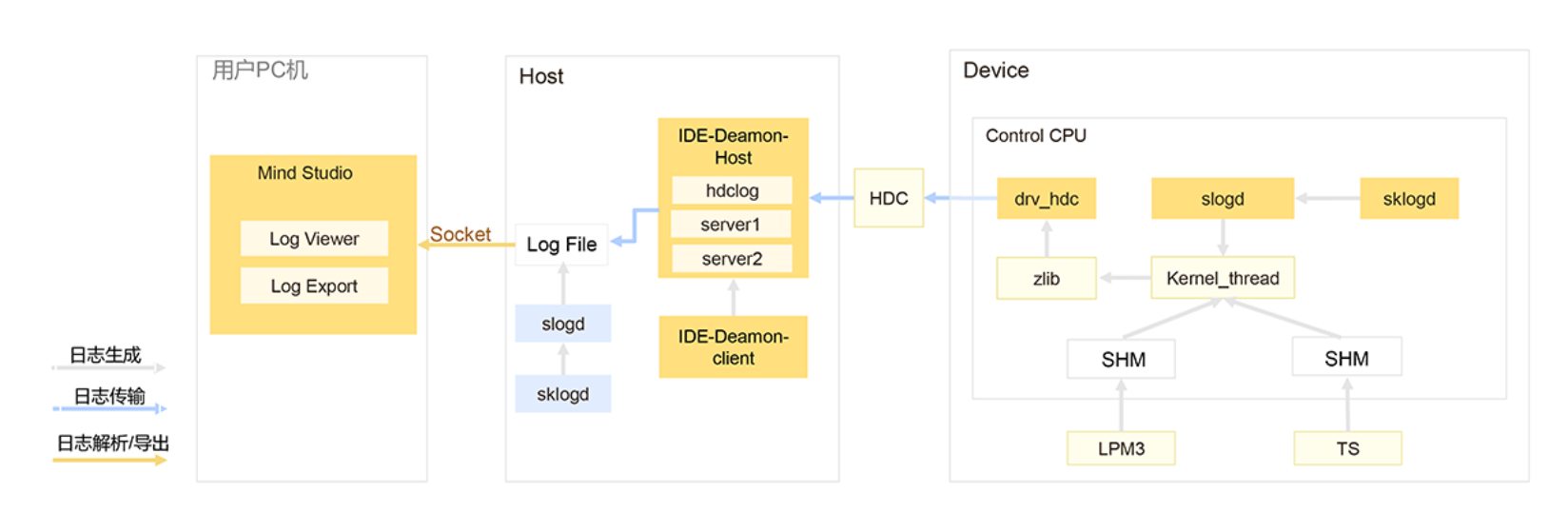全流程性能分析工具-profiling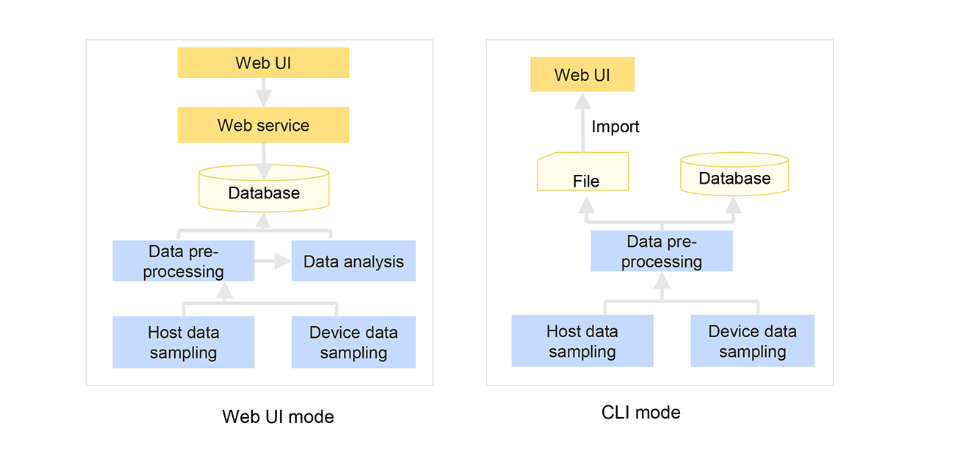比对工具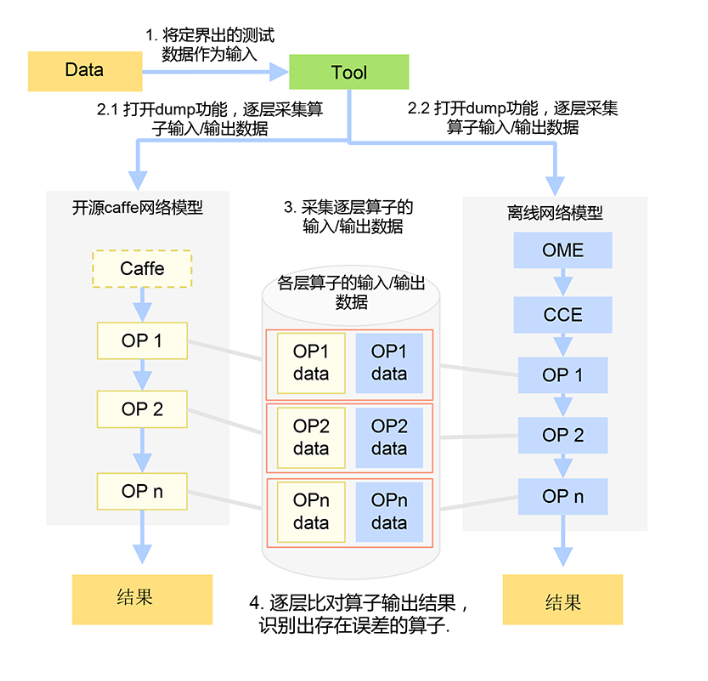二、TBE
TBE（Tensor Boost Engine）提供了昇腾AI处理器自定义算子开发能力，通过TBE提供的API和自定义算子编程开发界面可以完成相应神经网络算子的开发。TBE给用户提供了多层灵活的算子开发方式，用户可以根据对硬件的程度自由选择，利用工具的优化和代码生成能力，生成昇腾AI处理器的高性能可执行算子。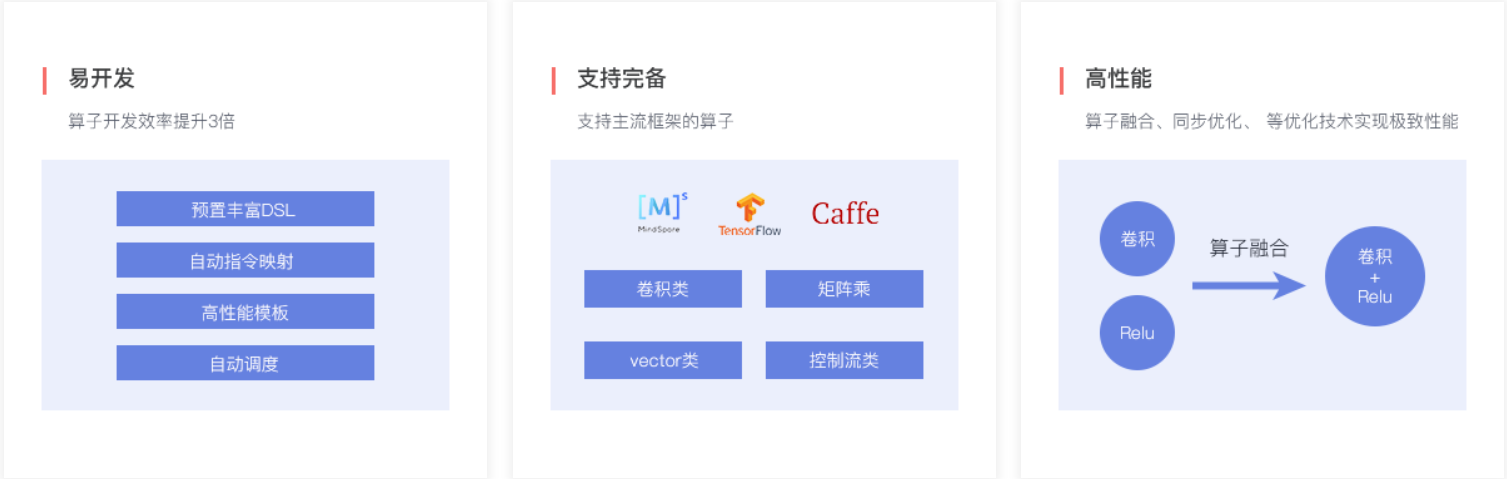参考：华为云社区


展开全文人工智能
• ## 华为机试题目笔记

千次阅读 2020-03-16 20:42:43
华为机试题目笔记服务器广播航班预定统计判断一组数连续九宫格输入抢票系统报数游戏字符串分割单词压缩编码分子弹/分发糖果打印任务排序集五福双列表元素分配 服务器广播 题目：服务器连接方式包括直接相连，间接...
华为机试题目笔记服务器广播航班预定统计判断一组数连续九宫格输入抢票系统报数游戏字符串分割单词压缩编码分子弹/分发糖果打印任务排序集五福双列表元素分配
服务器广播

题目：服务器连接方式包括直接相连，间接连接。 A 和 B 直接连接， B 和 c 直接连接，则 A 和 c 间接连接。直接连接和间接连接都可以发送广播。
给出一个 N * N 数组，代表 N 个服务器， matrix[i][j] == 1 ，则代表 i 和 j 直接连接；不等于 1 时，代表 i 和 j 不直接连接。 matrix[i][i]== 1 ，即自己和自己直接连接。 matrix[i][j]==matrix[j][i] 。计算初始需要给几台服务器广播，才可以使侮个服务器都收到广播。

输入描述： n * n 矩阵，
[[1,1,0],[1,1,0],[0,0,1]]

输出描述：整数
2

var broadcast = function(list) {
const count=[];// 存储能够连接的广播列表
for(let i=0;i<list.length;i++){
for(let j=i+1;j<list.length;j++){
if(list[i][j]==1){
if(count.length>0){
let flag = false;
count.map((item,index)=>{
if(item.includes(i)&&!item.includes(j)){
count[index].push(j);
}else if(item.includes(j)&&!item.includes(i)){
count[index].push(i);
}else if(!item.includes(i)&&!item.includes(j)){
flag=true;
}
})
if(flag){
count.push([i,j])
}
}else{
count.push([i,j])
}
}
}
}
for(let i=0;i<list.length;i++){
let flag=false;
count.map(item=>{
if(item.includes(i)){
flag=true
}
})
if(!flag){
count.push([i])
}
}
// count=[[0,1],]
return count.length
};

console.log(broadcast([[1,1,0],[1,1,0],[0,0,1]])) // 2

航班预定统计

题目：有 n 个航班，它们分别从 1 到 n 进行编号。
我们这儿有一份航班预定表，表中第 i 条预定记录 bookings[i]= [i , j , k ］意味着我们在从 i 到 j的每个航班上预订了 k 个座位。
请返回一个长度为 n 的数组answer ，按航班编号顺序返回每个航班上预订的座位数。
输入描述： 前几行为航班预定信息，最后一行为航班数量．

[[1 , 2 , 10], [2 , 3 , 20],[2 , 5 , 25]]
5

输出描述：长度为n的数组
[10,55,45,25,25]

var corpFlightBookings = function(bookings, n) {
const count = new Array(n).fill(0);
bookings.map(item=>{
count[item-1]+=item;
if(item<n){
count[item]-=item;
}
})
// count=[10,45,-10,-20,0]
return count.map((item,index)=>{
let sum=0;
while(index>=0){
sum+=count[index];
index--;
}
return sum;
})
};
console.log(corpFlightBookings([[1,2,10],[2,3,20],[2,5,25]])) //[10,55,45,25,25]


解题思路：

将问题转换为：某公交车共有 n 站，第 i 条记录 bookings[i] = [i, j, k] 表示在 i 站上车 k 人，乘坐到 j 站，在 j站下车，需要按照车站顺序返回每一站车上的人数
根据 1 的思路，定义 counter[] 数组记录每站的人数变化，counter[i] 表示第 i+1 站。遍历 bookings[]：bookings[i] = [i, j, k] 表示在 i 站增加 k 人即 counters[i-1] += k，在 j站减少 k 人即 counters[j] -= k
遍历（整理）counter[] 数组，得到每站总人数： 每站的人数为之前站人数变化加上当前人数变化

判断一组数连续

题目：给一个有序数组，如[1,2,3,5,7,9,10,12]，将连续的元素合并，中间用‘-‘连接，返回字符串。
输入描述：有序字符串，

[1,2,3,5,7,9,10,12]

输出描述：字符串
1-3，5，7，9-10，12

var sort = function(arr) {
const newArr=[];
for(let i=0;i<arr.length;){
let j=i;
while(arr[j]+1===arr[j+1]&&j<arr.length){
j++
}
if(i!==j){
newArr.push(${arr[i]}-${arr[j]});
i=j+1
}else{
newArr.push(arr[i])
i++;
}
}
return newArr.join(',')
};
// 1-3，5，7，9-10，12
console.log(sort([1,2,3,5,7,9,10,12]))

九宫格输入

题目：假设有九宫格输入法键盘布局如下：

 [ 1,.?! ] [ 2ABC ] [ 3DEF  ]
[ 4GHI  ] [ 5JKL ] [ 6MNO  ]
[ 7PQRS ] [ 8TUV ] [ 9WXYZ ]
[ 0空  ]

注意：中括号[ ]仅为了表示键盘的分隔，不是输入字符。每个中括号中，位于首位的数字字符即是键盘的按键，按一下即可输入该数字字符。多次按同一个键，则输入的字符依次循环轮流，例如按两次3，则输入D；按5次7，则输入S；按6次2，则输入A。按键0的输入组合是0和空格字符，即按两次0输入空格。
你需要对于给定的按键组合，给出该组合对应的文本。
输入格式:
输入在一行中给出数个字符的按键组合（例如 999 表示按3次9），每个字符的按键组合之间用空格间隔，最后一个输入法组合之后以换行结束。输入数据至少包括一个字符的按键组合，且输入总长度不超过500个字符。
['22', '5555', '22', '666', '00', '88', '888', '7777', '4444', '666', '44']

输出格式:
在一行中输出该按键组合对应的文本。
ALAN TURING

var nineInput = function(arr) {
const button=["0 ","1,.?!","2ABC","3DEF","4GHI","5JKL","6MNO","7PQRS","8TUV","9WXYZ"];
let str='';
for(let i=0;i<arr.length;i++){
let num=arr[i];
let index=(arr[i].length>button[num].length?(arr[i].length%button[num].length):arr[i].length)-1
str+=button[num][index]
}
return str
};

console.log(nineInput(['22', '5555', '22', '666', '00', '88', '888', '7777', '4444', '666', '44']))

抢票系统

题目：输入年月日时分秒，输出多少人能抢到票；
规则：
( 1 ）同一秒内，若时间不同，选最早的，若存在时间相同，选择多个；
( 2 ）不同时间，选择时间最早的；
输入描述： 时间数组

['1992 一 08 一 20 12 : 12 : 12 . 001 ','1992 一 08 一 20 12 : 12 : 12 . 003 ','1992 一 08 一 20 12 : 12 : 12 . 203 ','1992 一 08 一 21 12 : 12 : 12 . 001 ','1992 一 08 一 21 12 : 12 : 12 . 003']

输出描述：整数．
2



报数游戏

题目：有 n (1<n<10000)个小朋友站成一个圆圈。
选定一个小朋友为1号，从他（她）开始顺时针编号：1,2,3,4，…

游戏开始! 从1号小朋友起,顺时针报数,从1报起。
即：1号小朋友报1，2号小朋友报2，3号小朋友报3, …
游戏规定，报到数字 m(1<m<100) 的小朋友立即退出报数圈。
在他（她）的顺时针方向的下一个小朋友（如果有的话）开始重新从1报数…
游戏这样一直进行下去，直到圈中只剩下一个小朋友。
求最后剩下的小朋友的编号。
输入：两个整数，n 和 m。
输出：一个整数，表示最后剩下的小朋友的编号。
比如：
输入：
15 3
程序应该输出：
5
再比如：
输入：
7 4
程序应该输出：
2
var find = function(n,m) {
let arr=new Array(n).fill(true);
let count=0;// 数组下标
let index=0;// 报数记录
let total=n;// 已退出游戏人数
while(count<n&&total>1){
if(arr[count]){
if(index===m-1){
arr[count]=false;
index=0;
total--;
}else{
index++;
}
}
count++;
if(count===n){
count=0;
}
}
return arr.indexOf(true)+1
};

console.log(find(7,4))

字符串分割

题目：实现字符串分割算法split(str,delim),传入待分割字符串和分割字符，返回数组，如果delim参数不传，则对字符串逐个分割。
比如调用split(“abcbebb”,‘b’)，返回为[“a”,“c”,“e”]，调用split(‘abcd’)，返回[‘a’,‘b’,‘c’,d’]

var split=function(str,delim=''){
let newStr=[];
let temp=''
for(let i=0;i<str.length;){
if(delim.length>0){
let index=0;
while(index<delim.length&&str[i+index]===delim[index]){
index++;
}
if(index<delim.length){
temp+=str[i];
i++;
}else{
if(temp.length>0){
newStr.push(temp)
};
temp='';
i+=delim.length
}
}else{
newStr.push(str[i]);
i++;
}
}
if(temp.length>0){
newStr.push(temp)
}
return newStr;
}
// [a,b,c,e]
console.log(split('adbdcdedd','d'))

单词压缩编码

题目：给定一个单词列表，将这个列表编码成一个索引字符串 S 与一个索引列表 A。
例如，如果这个列表是 [“time”, “me”, “bell”]，将其表示为 S = “time#bell#” 和 indexes = [0, 2, 5]。
对于每一个索引，可以通过从字符串 S 中索引的位置开始读取字符串，直到 “#” 结束，来恢复之前的单词列表。
输入描述：数组。
输出描述：成功对给定单词列表进行编码的最小字符串长度

// 输入,说明index=[0,2,5],str='time#bell#'
['time','me','bell']
// 输出
10

var minimumLengthEncoding=function(words){
let newStr='';
let str=words.map(item=>{
let temp=''
for(let i=item.length-1;i>=0;i--){
temp+=item[i]
}
return temp;
}).sort();// 反转排序
for(let i=0;i<str.length;i++){
if(str[i+1]!==undefined){
if(str[i+1].indexOf(str[i])!==0){
newStr+=#${str[i]} } }else{ newStr+=#${str[i]}
}
}
return newStr.length;
}
console.log(minimumLengthEncoding(['time','me','bell']))

分子弹/分发糖果

题目：老师想给孩子们分发糖果，有 N 个孩子站成了一条直线，老师会根据每个孩子的表现，预先给他们评分。
你需要按照以下要求，帮助老师给这些孩子分发糖果：
每个孩子至少分配到 1 个糖果。
相邻的孩子中，评分高的孩子必须获得更多的糖果。
那么这样下来，老师至少需要准备多少颗糖果呢？
示例 1:

输入: [1,0,2]
输出: 5
解释: 你可以分别给这三个孩子分发 2、1、2 颗糖果。

示例 2:
输入: [1,2,2]
输出: 4
解释: 你可以分别给这三个孩子分发 1、2、1 颗糖果。
第三个孩子只得到 1 颗糖果，这已满足上述两个条件。

var candy = function(ratings) {
let arr=new Array(ratings.length).fill(1);
for(let i=1;i<ratings.length;i++){
if(ratings[i]>ratings[i-1]){
arr[i]=arr[i-1]+1;
}
}
for(let j=ratings.length-2;j>=0;j--){
if(ratings[j]>ratings[j+1]&&arr[j]<=arr[j+1]){
arr[j]=arr[j+1]+1;
}
}
let total=0;
for(let i=0;i<arr.length;i++){
total+=arr[i]
}
return total
};
console.log()candy([1,0,1])

打印任务排序

题目：某个打印机根据打印队列执行打印任务。打印任务分为九个优先级，分别采用数字1~9表示，数字越大优先级越高。打印机每次从队列头部取出第一个任务A，然后检查队列余下任务中有没有比A优先级更高的任务，如果有比A优先级高的任务，则将任务A放到队列尾部，否则执行任务A的打印。请编写一个程序，根据输入的打印队列，输出实际打印顺序

var print = function(ratings) {
let arr=new Array(ratings.length).fill(0);
let index=1;
while(Math.max(...ratings)>0){
arr[ratings.indexOf(Math.max(...ratings))]=index;
index++;
ratings[ratings.indexOf(Math.max(...ratings))]=0;
}
return arr
};
// [1,5,3,4,2] 感觉过分简单，可能是我理解错误？
console.log(print([3,1,2,2,3]))

集五福

题目：以0和1组成的长度为5的字符串代表每个人所得到的福卡，每一位代表一种福卡，1表示已经获得该福卡，单类型福卡不超过1张，随机抽取一个小于10人团队，求该团队最多可以集齐多少套五福？
输入描述：数组，即团队每个人的福卡拥有状态，输出描述：数字，即最多可以集齐的数量

var print = function(ratings) {
let arr=new Array(5).fill(0);
for(let i=0;i<ratings.length;i++){
for(let j=0;j<5;j++){
arr[j]+=parseInt(ratings[i][j])
}
}
return Math.min(...arr)
};
// 1,arr=[ 4, 1, 4, 4, 3 ]
console.log(print(['10011','10110','01111','10111','10000','00100']))

双列表元素分配

题目：有两个数组a,b，大小都为n,数组元素的值任意，无序；要求：通过交换a,b中的元素，使数组a元素的和与数组b元素的和之间的差最小。

var getMinusArray = function(a,b) {
let suma=0;
let sumb=0;
for(let i=0;i<a.length;i++){
suma+=a[i];
sumb+=b[i];
}
let min=Math.abs(suma-sumb);
for(let i=0;i<a.length;i++){
for(let j=0;j<b.length;j++){
let temp=0;
if(Math.abs(2*(b[j]-a[i])+suma-sumb)<min){
suma=suma-a[i]+b[j];
sumb=sumb-b[j]+a[i];
temp=a[i];
a[i]=b[j];
b[j]=temp;
min=Math.abs(suma-sumb)
}
}
}
return {a,b}
};
console.log(getMinusArray([1, 3, 5 ,49],[0,2,4,18]))



展开全文• 笔试总结——0310华为软笔C++ 题目3：N个广播站，站点之间有些有连接，有些没有。有连接的站点在接受到广播后会互相发送。给定一个N*N的二维数组matrix，数组的元素都是字符’0’或者’1’。matrix [ i ][ j ]= ‘1...c++
• 题目描述: 题目描述： 矩阵相乘 输入：两个N*N阶矩阵相乘 输出：结果矩阵 样例输入： {1, 2, 3}, {4, 5, 6}, {7, 8, 9} {1, 2, 3}, {4, 5, 6}, {7, 8, 9} 样例输出： ...int matrix(int **MatrixA
• 蛇形矩阵： 蛇形矩阵是由1开始的自然数依次排列成的一个矩阵上三角形。 例如，当输入5时，应该输出的...int matrix(int N) { int rowFvalue = 1; int colomnFvalue=1; int colomnimt = 1; //行前两个数的差值 for.算法 数据结构
• 五福 #include #include //集齐五福多少套 //给出0 1 序列 using namespace std; int fu(string &str){ ... int matrix={0}; //int people_num = 1; int fu_num = 1; for (auto i : str){ if (i ==C++
• Smart Matrix华为高端存储的架构，在V3时代应用在18500和18800系列上，在V5时代也应用在了6800上，所以在OceanStor V5中6800也是高端存储。Smart Matrix架构的特点可简单总结为以下四点：Active-Active后端全互联...
• Tsai笔记：C++学习随性笔记（5）—— 华为面试题目总结 一.在一个二维01矩阵中找到全为1的最大正方形，返回其面积。 如以下样例样例: 返回 4(2*2) 解题思想 先构造一个辅助矩阵tmp，把matrix矩阵的第一行和第一...
• 1. 矩阵转置（20分） 问题描述：  ...public String matrixTranspose (String inArr, int n) 【输入】inArr： 输入的字符矩阵  n： N*N矩阵的行数 【返回】转置后的字符矩阵
• 1 矩阵转置 ...void MatrixTranspose (const char *InArr, unsigned int n, const char *OutArray) 【输入】InArr： 输入的字符矩阵 n： N*N矩阵的行数 【输出】OutArray： 转置后的字符
• //矩阵乘法 #include using namespace std;...void matrix_multiply(int *m1, int*m2, int*r, int x, int y, int z) { for (int i = 0; i ;i++) for (int j = 0; j ;j++) for (int k = 0; k ;k++) {循环
• #include <stdio.h> #include <stdlib.h> /* 3 1 2 3 4 5 6 7 8 9 */ int main() { int n; scanf("%d",&n);... int matrix[n][n]; int i, j; int temp[n][n]; for(i=0; i&...c++
• 题目链接：maximal-rectangle import java.util.Arrays;... * Given a 2D binary matrix filled with 0's and 1's, * find the largest rectangle containing all ones and return its area. * */ public cljava 算法 leetcode
• // MatrixTranspose.cpp : 定义控制台应用程序的入口点。 // /* 矩阵转置（20分） 问题描述 将一个N*N矩阵的行列互换 要求实现函数 void MatrixTranspose (const char *InArr, unsigned int n, const char *...指针常量 常量指针
• 一、问题描述 二维数组转置 例：1 2 3  4 5 6 转置  1 4  2 5 ... * Gets reversed matrix * @param a * @return */ public int[][] reverseArray(int[][] a) { Integer[] rowCel
• 昇腾AI软件栈提供了计算资源、性能调优的运行框架以及功能...· 神经网络软件主要包含了流程编排器（Matrix），框架管理器（Framework），运行管理器（Runtime）、数字视觉预处理模块（Digital Vision Pre-Processi...人工智能 AI 华为云 处理器
• 用户可以使用标准的Matrix接口实现业务引擎，对外释放昇腾AI加速能力。 从卷积神经网络中的矩阵乘法（GEMM）说起 说起AI业务，就不得不提最经典的AlexNet，AlexNet模型于2012年提出，其被认为是计算机视觉领域最...atlas 人工智能 AI 华为云
• 问题：数组的螺旋遍历，逻辑模拟，主要技巧在于四个方向的控制。 一、螺旋矩阵(leetcode54) 问题： 题目来源：力扣（LeetCode） 链接：... def spiralOrder(self, matrix: List[List[ileetcode python 算法 动态规划
• Matrix是已经支持phthon接口了，但是发现目前python的hiai库并没有自动安装，需要自己安装； 话不多说下面是安装步骤： 步骤1. 开发板联网，如果已联网则跳过该步骤；  否则请参考以下链接配置开发板联网...
• 既然Matrix提供了python接口，那咱们就要将方便的用起来，接下来分享一个利用pycharm直接搞定开发板开发环境的方法；并以开源的一个pythondemo来说明如何玩起来（案例地址：...华为云 Atlas python 开发
• 它是一个典型的动态规划问题，同时也是2017京东面试题，2016华为机考题。题目描述　有一个n*m大小的矩阵，其元素值为0或者1，求这个矩阵中全有1组成的最大方块其大小。输入描述　每个输入包含一个测试用例。每个测试...面试题 动态规划
• 最近使用Matrix遇到一件比较诡异的问题，使用 Matrix.setPolyToPoly 接口绘制一个多边形，在 华为 手机上遇到变形错位的问题. 把 Matrix 作用到系统传递过来的 canvas 上面，理论上来说这应该是没有问题的，但...Android 硬件加速 多边形
• 11月30日消息，继华为Mate 30系列后，荣耀在11月26日也带来了双模5G手机荣耀V30。值得一提的是，这款荣耀V30不但有行业领先的双模5G功能，还有全新的Matrix Came...
• Maximal Square----动态规划–华为，谷歌面试算法题–Python解法 LeetCode题解专栏：LeetCode题解 我做的所有的LeetCode的题目都放在这个专栏里，大部分题目C++和Python的解法都有。 题目地址：Maximal Square - ...
• 输入一个矩阵，按照从外向里以顺时针的顺序依次打印出每一个数字。 示例 1： 输入：matrix = [[1,2,3],[4,5,6],[7,8,9]] ...1.经典题，华为笔试改编 2.弄成魔方旋转来做 class Solution: def spiralOrder(sel..